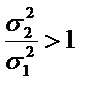You are here (click or tap to change)

# Problem 1

9.10 Using the f-table to Find Critical Values

Use the f-table to find the critical value for each of the given scenario:

Sample 1: variance = 37, n1 = 14  Sample2: variance = 89, n2 = 25

Claim:Significance Level: 0.01